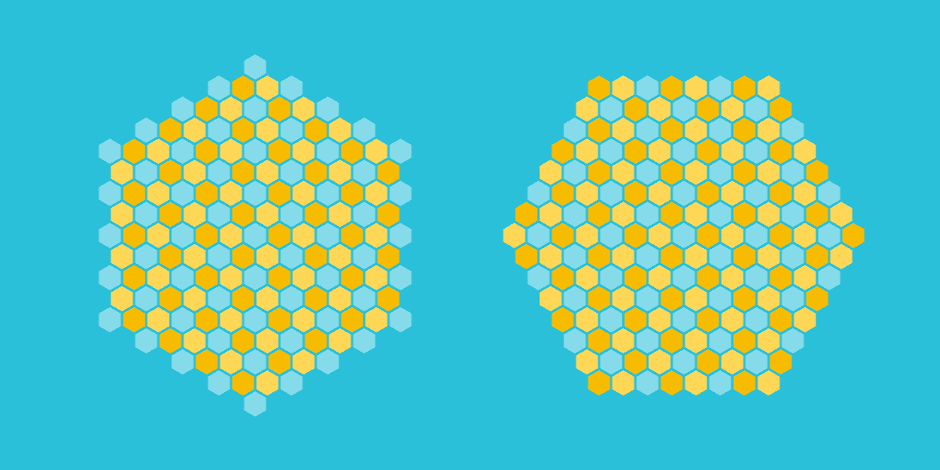Pointy hex grids have built-in support for flat grid shapes, as shown on the right above. However, there is no support for pointy hex shapes, and we did not even think such shapes could really be made nicely until one of our customers asked us how to make them. As you can see above, they can be made.

The code is very short, but a bit tricky to understand. Essentially, the formula is the same as that for the flat hex shape, but rotated by 30 degrees. To derive the formula for a 30 degrees turn, we take the square root of the matrix M that rotates a point by 60 degrees. This gives a matrix with non-integer values. Fortunately, we can multiply with a constant factor (2 / sqrt(3)) to have integers points, as long as we modify our radius of the check accordingly.

Up to this point we also get some other pointy shapes, such as the one below. They fall between the “right” ones, and we can eliminate them by some more scaling and offsetting – this is the (3.5f * size – 3) factor, which we determined with trial and error.

PointyHexShape.unitypackage